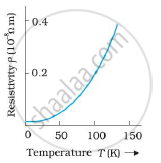Share

# Show Variation of Resistivity of Copper as a Function of Temperature in a Graph. - CBSE (Science) Class 12 - Physics

ConceptElectrical Resistivity and Conductivity

#### Question

Show variation of resistivity of copper as a function of temperature in a graph.

#### Solution

The variation of resistivity of copper with temperature is parabolic in nature. This is shown in the following graph:

Temperature V5 resistivity graph of a copper
resistivity of copper increases with incresase in tempratureIs there an error in this question or solution?

#### Video TutorialsVIEW ALL 

Solution Show Variation of Resistivity of Copper as a Function of Temperature in a Graph. Concept: Electrical Resistivity and Conductivity.
S